# Understanding Your Neural Network’s Predictions

Deep LearningModelingposted by ODSC Community November 11, 2022

Neural networks are extremely convenient. They are usable for both regression and classification, work on structured and unstructured data, handle temporal...Neural networks are extremely convenient. They are usable for both regression and classification, work on structured and unstructured data, handle temporal data very well, and can usually reach high performances if they are given a sufficient amount of data.

What is gained in convenience is, however, lost in interpretability and that can be a major setback when models are presented to a non-technical audience, such as clients or stakeholders.

For instance, last year, the Data Science team I am part of wanted to convince a client to go from a decision tree model to a neural network, and for good reasons: we had access to a large amount of data and most of it was temporal. The client was on board, but wanted to keep an understanding of what the model based its decisions on, which means evaluating its features’ importance.

## Does it make sense?

That is debatable. With a decision tree or a boosting model, the features’ importance can be directly retrieved with the fitted attribute `feature_importances_` for most decision trees or the `get_booster()` and `get_score()`methods for XGBoost models.

For a neural network, these attributes and methods do not exist. Each neuron is trained to learn when to activate or not based on the signal it receives, so that each layer extracts some information — or concept — from the original input, up until the final prediction layer. Therefore, the usefulness of retrieving the features’ importance of a more “black-box” kind of model is questionable.

I’ve even heard deep learning experts say that it is best to let the data do the talking, and not to try to understand the model too much. Basically, is it useful to know whether a cat’s fur is more impactful for the neural networks than its eyes? Maybe not. But it is useful to know that, for the model, a cat on a table is no less a cat than one on the floor, and that’s what we’ll do here.

## Permutate, pertubate, and evaluate

We’ll use the permutation importance method. For classic machine learning models, Scikit-Learn provides a function to do that, and even recommends it when dealing with high cardinality features. If you want to use this function on your model, this code snippet will compute and display its permutation importance :

```import matplotlib.pyplot as plt
from sklearn.ensemble import RandomForestClassifier
from sklearn.impute import SimpleImputer
from sklearn.inspection import permutation_importance
from sklearn.compose import ColumnTransformer
from sklearn.pipeline import Pipeline
from sklearn.preprocessing import OneHotEncoder

# Separate numeric features from categorical features
numerical_columns = df.drop(columns="Target").select_dtypes(include = np.number).columns
categorical_columns = list(set(df.drop(columns="Target").columns) - \
set(numerical_columns))

# Prepare X (data) and Y (target)
X = df.dropna()[numerical_columns + categorical_columns]
Y = df.dropna()["Target"]

# Machine Learning Pipeline
categorical_encoder = OneHotEncoder(handle_unknown='ignore')
numerical_pipe = Pipeline([
('imputer', SimpleImputer(strategy='mean'))
])

preprocessing = ColumnTransformer(
[('cat', categorical_encoder, categorical_columns),
('num', numerical_pipe, numerical_columns)])

rf = Pipeline([
('preprocess', preprocessing),
('classifier', RandomForestClassifier(random_state=42))
])

# Model fit
rf.fit(X, Y)

# Plot
result = permutation_importance(rf, X, Y, n_repeats=10,
random_state=42, n_jobs=2)
sorted_idx = result.importances_mean.argsort()

fig, ax = plt.subplots(figsize = (18, 20))
ax.boxplot(result.importances[sorted_idx].T,
vert=False, labels=X.columns[sorted_idx])

plt.suptitle(
"Importance of a random permutation of a feature on the model's outputs",
fontsize = 16
)

plt.show()```

## The principle behind permutation importance

Let’s say you have several students, and you want to evaluate their likelihood of passing a math exam. To do so, you have access to 3 variables : the time they spent studying for the exam, their ease in math, and their hair color.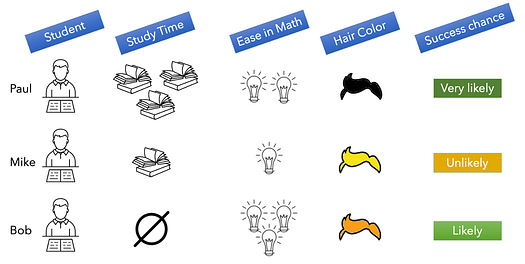Student data for a math exam. Image by author

In this example, Paul studied a lot, and is moderately gifted in math. He is very likely to succeed to his math exam. Mike, on the other hand, studied much less and is not very gifted, he is unlikely to succeed. Bob didn’t study at all, but is extremely gifted, he therefore has his chances.

Let’s permutate the values of the “Study Time” feature: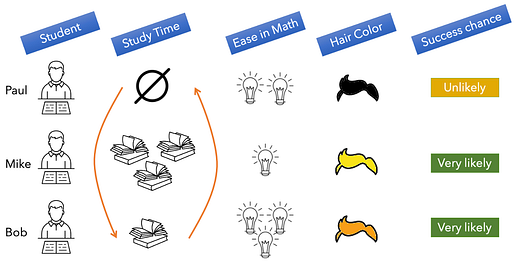Impact of shuffling the 1st column. Image by author

Paul went from studying a lot to not studying at all. His moderate ease in math will not be enough to compensate, and he is now unlikely to pass. Likewise, the other students had their likelihood of success highly impacted by this perturbation.

We can therefore infer that the study time is an important feature to predict the exam’s outcome.

We get the same result when we perturbate the ease in math feature :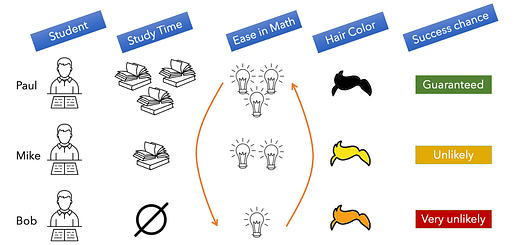Impact of shuffling the 2nd column. Image by author

Bob has now become ungifted in math, and hasn’t studied at all. It is extremely unlikely that he passes the exam.

With the same reasoning as before, this feature is also important.

Now, when we permutate the hair color feature :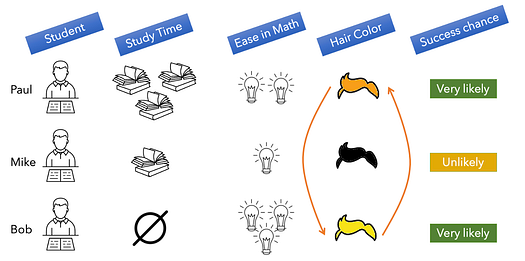Impact of shuffling the 3rd column. Image by author

Mike’s going from blond to dark haired will not improve his chances for the exam, nor will any hair color change will have any impact on any student. This feature therefore has no importance on our prediction.

## Limits of this method

Let’s say that out of 100 students, we have one cheater that managed to get his hand on the test subject, which guarantees him a pass on the exam. If we permutate the “cheater” column, we’ll have only one student going from cheater to non-cheater, and one other student that goes from non-cheater to cheater. Out of 100 students, only two will be impacted, and we’ll wrongfully consider this feature as unimportant because of its low prevalence.

Therefore, this method will not work well on unbalanced binary features and on rare modalities of categorical features. For these cases, it is better to set the whole column to the rare value and see how it impacts the prediction (in our analogy, that would mean setting the “cheater” column to True for every student).

## Implementation

The first step is to make an unperturbated inference on your testing set. Then, for each feature, you’ll shuffle it randomly and make what I’ll call a perturbated inference.

Once all the perturbated inferences are made, concatenate them in a single dataframe, and then calculate, for each observation, how far each of them has deviated compared to the original prediction.

From there, a good way to visualize the impact of each perturbation is to make a box-plot of all the observations’ deviations.

Let’s use, for instance, the Kaggle dataset for the Home Credit Default Risk competition.
After the pre-processing and training stages, I got two datasets,`X_test` which contains the static data for the testing set and `X_test_batch` which contains the temporal data for the training set.

The following snippet goes through every feature and creates a perturbated inference :

```import numpy as np
import pandas as pd
import os
import tensorflow as tf
from tensorflow.keras.layers import Input, Embedding, Reshape, Dense, BatchNormalization, Masking, LSTM
from tensorflow.keras.models import Model

# numcols is a list of static numeric features
# catcols is a list of static categorical features
# temporal_features is a list of temporal features
# input_path is the folder where the data and model weights are stores
# output_path is the folder where the perturbated inferences will be stored
static_features = numcols + catcols

static_data = X_test.copy()

def make_inference(inference_name, static_data, temporal_data, numcols, catcols):
test_dict = {}
for categorical_var in catcols:
keyname = categorical_var + "_input"
test_dict[keyname] = static_data[categorical_var]
test_dict["num_input"] = static_data[numcols]
test_dict["temporal_input"] = temporal_data
nn_pred = model.predict(test_dict, batch_size = 3000)

df = pd.DataFrame()
colname = "inference_{}_perturbated".format(inference_name)
df[colname] = nn_pred.squeeze()

df.to_csv(os.path.join(output_path, "inference_{}_perturbated.csv".\
format(inference_name)))

# Make perturbated inferences for static features
static_data = X_test.copy()
for feature in static_features:
if os.path.exists(os.path.join(output_path, "inference_{}_perturbated.csv".\
format(feature))):
continue
print("handling feature {}".format(feature))
# Shuffle feature
static_data[feature] = static_data[feature].sample(frac=1).values
# Infer
make_inference(inference_name=feature,
static_data=static_data,
temporal_data=X_test_batch,
numcols=numcols,
catcols=catcols)
# Undo perturbation
static_data[feature] = X_test[feature]

# Make perturbated inferences for temporal features
temporal_data = X_test_batch.copy()
for idx, feature in enumerate(temporal_features):
if os.path.exists(os.path.join(output_path, "inference_{}_perturbated.csv".\
format(feature))):
continue
print("handling feature {}".format(feature))
# Shuffle feature
temporal_data[:, idx, :] = np.take(temporal_data[:, idx, :],
np.random.permutation(temporal_data[:, idx, :].\
shape),
axis=0)
# Infer
make_inference(inference_name=feature,
static_data=X_test,
temporal_data=temporal_data,
numcols=numcols,
catcols=catcols)
# Undo perturbation
temporal_data[:, idx, :] = X_test_batch[:, idx, :]```

Then, this code snippet will compute the deviation from the original inference for each perturbation :

```import pandas as pd
import numpy as np
import os
import re

index_col=0).reset_index(drop=True)
all_perturbations.rename(columns = {"inference" : "orginal_inference"}, inplace=True)
print(all_perturbations.shape)

pattern = r"\s*inference_.*"
toread_list = [f for f in os.listdir(perturbated_inferences_path) \
if re.search(pattern, f)]

# Concatenation loop
index_col=0).reset_index(drop=True)
all_perturbations = pd.concat([all_perturbations, tempdf], axis=1)
print("shape", all_perturbations.shape)

# Check NaNs
print("nans", all_perturbations.isna().sum().sum())

score_cols = [colname for colname in all_perturbations.columns \
if "inference" in colname and colname != "orginal_inference"]

def get_perturb_name(text):
match = re.search('inference(.+)', text)
if match:
found = match.group(1)
return(found)

# Get perturbation impact compared to original inference
output_df = pd.DataFrame()
for colname in score_cols:
new_colname = "PERT_" + get_perturb_name(colname)
output_df[new_colname] = (all_perturbations["orginal_inference"] - \
all_perturbations[colname]).\
map(lambda X: abs(X))

# Write recipe outputs
output_df.to_csv("perturbation_impact.csv")```

Finally, this code snippet will print the feature importance :

```import pandas as pd
import numpy as np
import matplotlib.pyplot as plt
import seaborn as sns
import os

# output_path is the folder where the plot will be saved

def q50(x):
return np.quantile(x, 0.5)

dfq50 = df.apply(q50, axis=0)
maxvalue = df.max(axis=0)
maxvalue = maxvalue.max()

tidy = df.melt().rename(columns=str.title)

fig, ax = plt.subplots(figsize=(18,60))

sns.boxplot(data = tidy,
y="Variable",
x="Value",
orient="h",
showfliers=False,
order=dfq50.sort_values(ascending=False).index,
ax=ax)

ax.hlines(19.525,
0,
maxvalue,
color="r",
linestyle="--",
label="TOP 20 FEATURES",
linewidth=2)

plt.legend(fontsize = 18)

axsuptitle = "Impact of a random shuffle in a column on the inference"

ax.set_title(axsuptitle,
fontsize = 18,
fontweight="bold")

ax.set_xlabel("Difference between original inference and perturbated inference",
fontsize = 14,
fontweight = "bold")

ax.set_ylabel("Perturbated feature",
fontsize = 14,
fontweight = "bold")

plt.style.use("bmh")

plt.gca().set_facecolor("white")

plt.setp(ax.spines.values(), color='k')

fig.tight_layout()

plt.savefig(os.path.join(output_path, "feature_importance.png"))```

You should get a plot like this :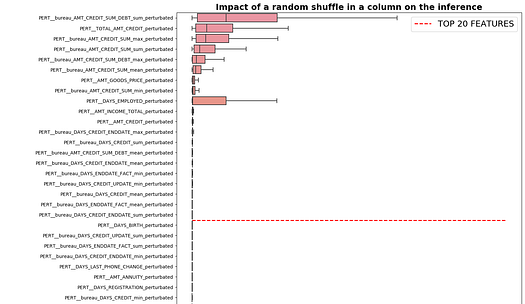If you want to see the whole data science pipeline, I have made a public docker image that contains all of the steps from the raw data up to the feature importance plot here : https://hub.docker.com/r/villatteae/neuralnet_feat_importance/tags

Simply run the following docker commands :

docker pull villatteae/neuralnet_feat_importance:latest

```docker pull villatteae/neuralnet_feat_importance
docker run -p 10000:10000 -d villatteae/neuralnet_feat_importance```